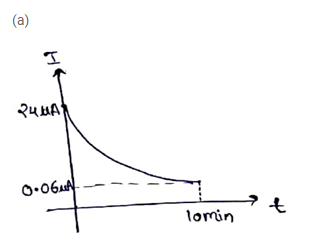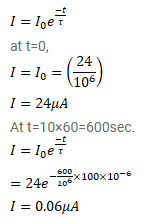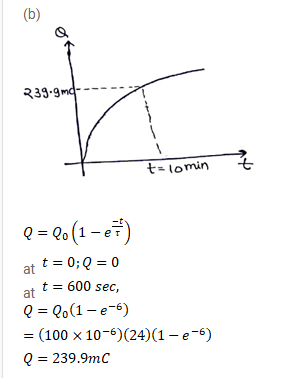# A 100μF capacitor is joined to a 24V battery through a 1.0MΩ resistor.

Question:

A $100 \mu \mathrm{F}$ capacitor is joined to a $24 \mathrm{~V}$ battery through a $1.0 \mathrm{M} \Omega$ resistor. Plot qualitative graphs

(a) Between current and time for the first 10 minutes and

(b) Between charge and time for the same period

Solution: